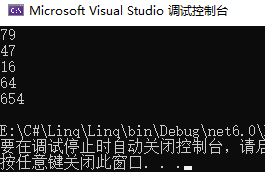# 揭秘Linq方法的背后

## 1. Linq方法

``````static void Main(string[] args)
{
int[] nums = { 10, 2, 5, 6, 79, 47, 16, 4, 1, 64, 654 };
IEnumerable<int> list = nums;
IEnumerable<int> result = list.Where(x => x > 10);
foreach (var item in result)
{
Console.WriteLine(item);
}
}
``````

``````bool f(int i)
{
return i>10;
}
``````

## 2. 创建自己的Where方法

``````static IEnumerable<int> MyWhere(IEnumerable<int> list,Func<int,bool> f)
{
List<int> result = new List<int>();
foreach (var item in list)
{
if (f(item))
{
}
}
return result;
}
``````## 3. Where方法的另一种实现方法

``````static IEnumerable<int> MyWhere(IEnumerable<int> list, Func<int, bool> f)
{
foreach (var item in list)
{
if (f(item))
{
yield return item;
}
}
}
``````